## Solutions to Try Its

1. $\left(-\frac{1}{2},\frac{1}{2}\right)$ and $\left(2,8\right)$

2. $\left(-1,3\right)$

3. $\left\{\left(1,3\right),\left(1,-3\right),\left(-1,3\right),\left(-1,-3\right)\right\}$

4. Shade the area bounded by the two curves, above the quadratic and below the line.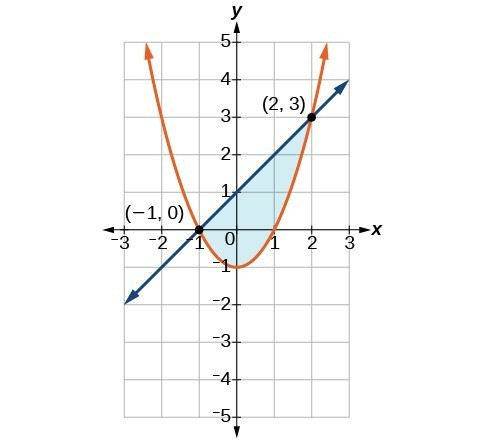## Solutions to Odd-Numbered Exercises

1. A nonlinear system could be representative of two circles that overlap and intersect in two locations, hence two solutions. A nonlinear system could be representative of a parabola and a circle, where the vertex of the parabola meets the circle and the branches also intersect the circle, hence three solutions.

3. No. There does not need to be a feasible region. Consider a system that is bounded by two parallel lines. One inequality represents the region above the upper line; the other represents the region below the lower line. In this case, no points in the plane are located in both regions; hence there is no feasible region.

5. Choose any number between each solution and plug into $C\left(x\right)$ and $R\left(x\right)$. If $C\left(x\right)<R\left(x\right),\text{}$ then there is profit.

7. $\left(0,-3\right),\left(3,0\right)$

9. $\left(-\frac{3\sqrt{2}}{2},\frac{3\sqrt{2}}{2}\right),\left(\frac{3\sqrt{2}}{2},-\frac{3\sqrt{2}}{2}\right)$

11. $\left(-3,0\right),\left(3,0\right)$

13. $\left(\frac{1}{4},-\frac{\sqrt{62}}{8}\right),\left(\frac{1}{4},\frac{\sqrt{62}}{8}\right)$

15. $\left(-\frac{\sqrt{398}}{4},\frac{199}{4}\right),\left(\frac{\sqrt{398}}{4},\frac{199}{4}\right)$

17. $\left(0,2\right),\left(1,3\right)$

19. $\left(-\sqrt{\frac{1}{2}\left(\sqrt{5}-1\right)},\frac{1}{2}\left(1-\sqrt{5}\right)\right),\left(\sqrt{\frac{1}{2}\left(\sqrt{5}-1\right)},\frac{1}{2}\left(1-\sqrt{5}\right)\right)$

21. $\left(5,0\right)$

23. $\left(0,0\right)$

25. $\left(3,0\right)$

27. No Solutions Exist

29. No Solutions Exist

31. $\left(-\frac{\sqrt{2}}{2},-\frac{\sqrt{2}}{2}\right),\left(-\frac{\sqrt{2}}{2},\frac{\sqrt{2}}{2}\right),\left(\frac{\sqrt{2}}{2},-\frac{\sqrt{2}}{2}\right),\left(\frac{\sqrt{2}}{2},\frac{\sqrt{2}}{2}\right)$

33. $\left(2,0\right)$

35. $\left(-\sqrt{7},-3\right),\left(-\sqrt{7},3\right),\left(\sqrt{7},-3\right),\left(\sqrt{7},3\right)$

37. $\left(-\sqrt{\frac{1}{2}\left(\sqrt{73}-5\right)},\frac{1}{2}\left(7-\sqrt{73}\right)\right),\left(\sqrt{\frac{1}{2}\left(\sqrt{73}-5\right)},\frac{1}{2}\left(7-\sqrt{73}\right)\right)$

39.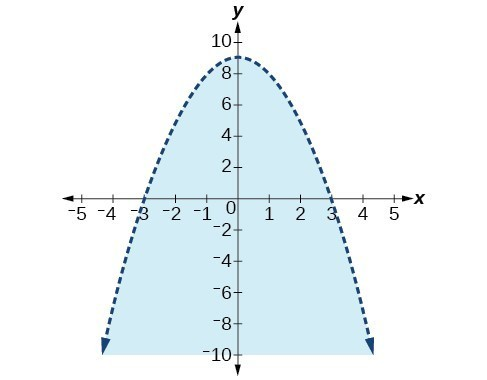41.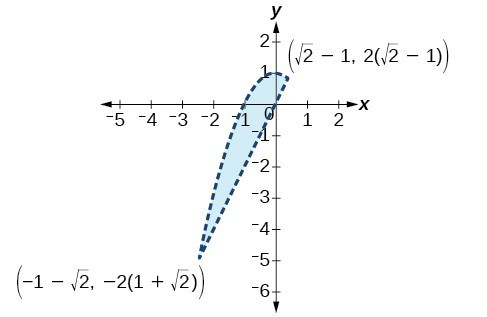43.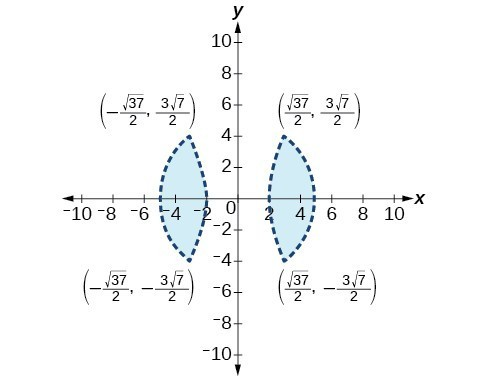45.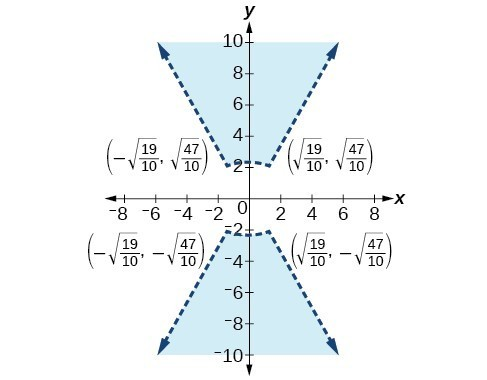47.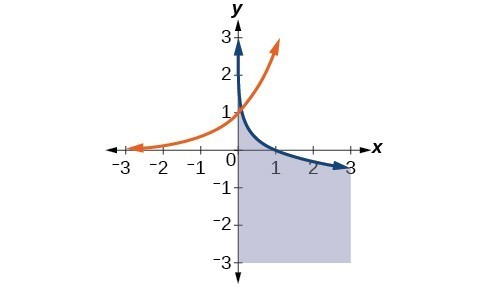49. $\left(-2\sqrt{\frac{70}{383}},-2\sqrt{\frac{35}{29}}\right),\left(-2\sqrt{\frac{70}{383}},2\sqrt{\frac{35}{29}}\right),\left(2\sqrt{\frac{70}{383}},-2\sqrt{\frac{35}{29}}\right),\left(2\sqrt{\frac{70}{383}},2\sqrt{\frac{35}{29}}\right)$

51. No Solution Exists

53. $x=0,y>0$ and $0<x<1,\sqrt{x}<y<\frac{1}{x}$

55. 12, 288

57. 2–20 computers